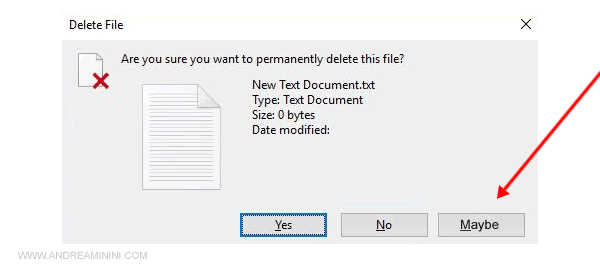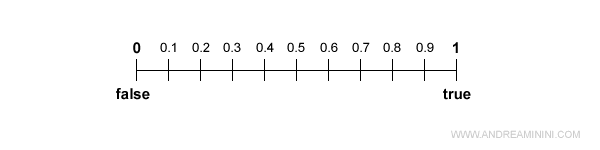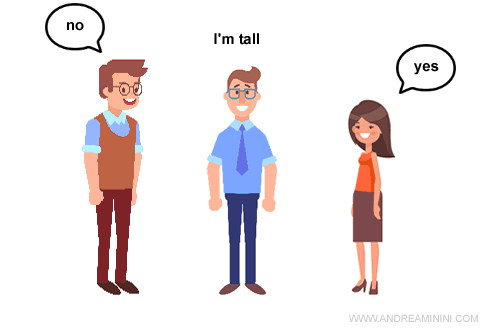# What's Fuzzy Logic

Fuzzy logic is a "nuanced" logic with infinite intermediate states between zero and one.

Example. In the fuzzy logic a variable can take the value 0 and 1 but also 0.2, 0.55, 0.8, etc. The fuzzy variable is a continuous variable and can assume infinite values between zero and one.

The fuzzy logic is the evolution of Boolean logic and it is particularly useful in the study of artificial intelligence.

## How fuzzy logic works

In computer science we usually use the Boolean logic which admits only two values: zero and one.

All programming languages are based on the binary logic of George Boole. A condition can be true or false, with no middle ground.

No programmer would ever develop a user interface with the yes, no, and maybe buttons.It is completely illogical to insert a third option ( Maybe ) between Yes and No to delete a file.

However, in artificial intelligence it becomes very useful.

Why is it useful?

Fuzzy logic allows computers to better represent the complexity of reality and the natural language of men.

### A practical example

In this photo the sky is clear or cloudy?In the Boolean logic I can choose between 0 (clear sky) and 1 (cloudy sky).

I could say that the sky is clear ... but it is not completely true because the sky is also a little cloudy.

Note. Boolean logic has a limited scope because reality is much more complex. In the Boolean logic the previous information ("a little cloudy") can not be transformed into data.

In the fuzzy logic, however, zero and one are only the extremes of a continuous variable. The variable can also take intermediate values such as 0.9, 0.5, 0.1, etc ...Thanks to the fuzzy logic I can assign to the variable the value 0.3 in a scale between 0 (clear sky) and 1 (cloudy sky).

In practice, I say that the sky is basically serene but also a little cloudy.

In doing so, I better represent the real situation.

### Another practical example

In natural language, many human expressions are subjective and relative.

A Boolean machine could not understand them without falling into logical contradictions.

For example, if I say "I'm tall", what am I saying? There is no objective and absolute measure to define the height of something, it is a subjective and relative concept.In fuzzy logic I can transform subjective information into an objective fact.

Example

A basketball team has 7 players with different heights.

I can not say whether each of them is tall or short. So I use fuzzy logic.

• I order the players from highest to lowest.
• Then I associate the value one (1) to the highest and the value zero (0) to the lowest.

This scale allows me to assign percentages at intermediate heights.

For example, the fourth person is exactly in the middle of the scale, so the fuzzy variable has a value of 0.5 (or 50%). And so on.

Note. Thanks to the fuzzy logic I can affirm that the degree of truth of the statement "the boy is tall" is true to 0.5 for the fourth person. In the same way, I can affirm that even the statement "the boy is short" has a degree of truth equal to 0.5. In his group the boy is at the same time a little tall and a little short.

## Fuzzy logic and artificial intelligence

Fuzzy logic is very useful in artificial intelligence because it allows the rational agent to choose under conditions of uncertainty, using a nuanced and probabilistic representation of reality.

Apparently the system may seem weaker, because it is without certainty. In reality, it is considerably more powerful because it manages to deal with logical reasoning, avoiding the logical inconsistencies typical of human reasoning.

In many cases it is impossible to solve a problem in its entirety with the classical Boolean logic, due to computational reasons (processing time, amount of memory, etc.) or lack of information (imperfect information).

Instead, through the fuzzy logic it is possible.

The application of fuzzy logic to artificial neural networks is one of the most interesting fields of study, one that I think can make a significant contribution in the development of A.I.

Segnalami un errore, un refuso o un suggerimento per migliorare gli appuntiArtificial Intelligence